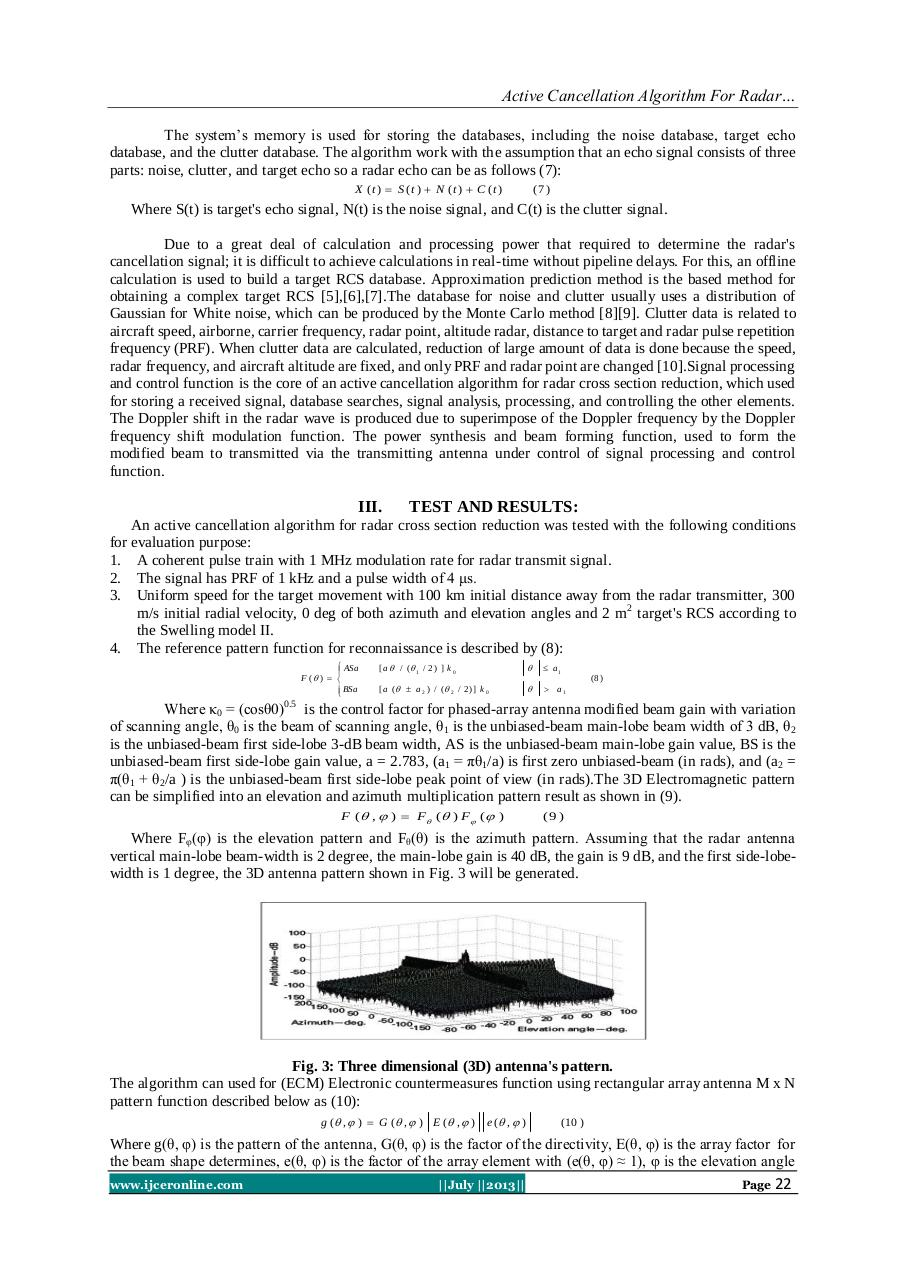# D0371019024.pdfPage 1 2 3 4 5 6

#### Text preview

The system’s memory is used for storing the databases, including the noise database, target echo
database, and the clutter database. The algorithm work with the assumption that an echo signal consists of three
parts: noise, clutter, and target echo so a radar echo can be as follows (7):
X (t )  S (t )  N (t )  C (t )

(7 )

Where S(t) is target's echo signal, N(t) is the noise signal, and C(t) is the clutter signal.
Due to a great deal of calculation and processing power that required to determine the radar's
cancellation signal; it is difficult to achieve calculations in real-time without pipeline delays. For this, an offline
calculation is used to build a target RCS database. Approximation prediction method is the based method for
obtaining a complex target RCS ,,.The database for noise and clutter usually uses a distribution of
Gaussian for White noise, which can be produced by the Monte Carlo method . Clutter data is related to
frequency (PRF). When clutter data are calculated, reduction of large amount of data is done because the speed,
radar frequency, and aircraft altitude are fixed, and only PRF and radar point are changed .Signal processing
and control function is the core of an active cancellation algorithm for radar cross section reduction, which used
for storing a received signal, database searches, signal analysis, processing, and controlling the other elements.
The Doppler shift in the radar wave is produced due to superimpose of the Doppler frequency by the Doppler
frequency shift modulation function. The power synthesis and beam forming function, used to form the
modified beam to transmitted via the transmitting antenna under control of signal processing and control
function.

III.

TEST AND RESULTS:

An active cancellation algorithm for radar cross section reduction was tested with the following conditions
for evaluation purpose:
1. A coherent pulse train with 1 MHz modulation rate for radar transmit signal.
2. The signal has PRF of 1 kHz and a pulse width of 4 μs.
3. Uniform speed for the target movement with 100 km initial distance away from the radar transmitter, 300
m/s initial radial velocity, 0 deg of both azimuth and elevation angles and 2 m 2 target's RCS according to
the Swelling model II.
4. The reference pattern function for reconnaissance is described by (8):
 ASa
F ( )  
 BSa

[ a  / ( 1 / 2 ) ] k 0

 a1

[ a (  a 2 ) / ( 2 / 2 )] k 0

 a1

(8 )

Where κ0 = (cosθ0)0.5 is the control factor for phased-array antenna modified beam gain with variation
of scanning angle, θ0 is the beam of scanning angle, θ1 is the unbiased-beam main-lobe beam width of 3 dB, θ2
is the unbiased-beam first side-lobe 3-dB beam width, AS is the unbiased-beam main-lobe gain value, BS is the
unbiased-beam first side-lobe gain value, a = 2.783, (a1 = πθ1/a) is first zero unbiased-beam (in rads), and (a2 =
π(θ1 + θ2/a ) is the unbiased-beam first side-lobe peak point of view (in rads).The 3D Electromagnetic pattern
can be simplified into an elevation and azimuth multiplication pattern result as shown in (9).
F ( ,  )  F  ( ) F  (  )

(9 )

Where Fφ(φ) is the elevation pattern and Fθ(θ) is the azimuth pattern. Assuming that the radar antenna
vertical main-lobe beam-width is 2 degree, the main-lobe gain is 40 dB, the gain is 9 dB, and the first side-lobewidth is 1 degree, the 3D antenna pattern shown in Fig. 3 will be generated.

Fig. 3: Three dimensional (3D) antenna's pattern.
The algorithm can used for (ECM) Electronic countermeasures function using rectangular array antenna M x N
pattern function described below as (10):
g ( ,  )  G ( ,  ) E ( ,  )

e ( ,  )

(10 )

Where g(θ, φ) is the pattern of the antenna, G(θ, φ) is the factor of the directivity, E(θ, φ) is the array factor for
the beam shape determines, e(θ, φ) is the factor of the array element with (e(θ, φ) ≈ 1), φ is the elevation angle
www.ijceronline.com

||July ||2013||

Page 22